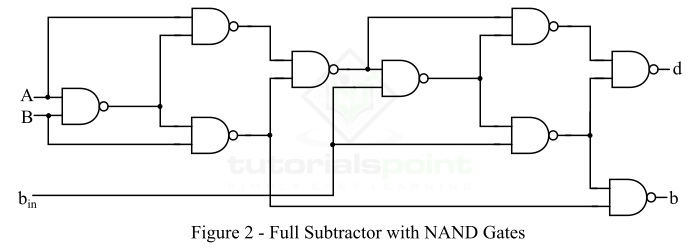# Realization of a Full Subtractor using NAND gate

In digital electronics, a subtractor is a combinational logic circuit that performs the subtraction of two binary numbers. However, the subtraction of binary number can be performed using adder circuits by taking 1s or 2s compliments. But, we may also realize a dedicate circuit to perform the subtraction of two binary numbers.

In the subtraction of two binary numbers, each subtrahend bit of the number is subtracted from its corresponding significant minuend bit to form a difference bit. During the subtraction, if the minuend bit is smaller than the subtrahend bit, then a 1 is borrowed from the next position. Depending upon the number of bits taken as input, there are two types of subtractors namely, Half Subtractor and Full Subtractor.

A half subtractor is one that takes two binary digits as input and gives a difference bit and a borrow bit (if any) as output. On the other hand, a full subtractor is one that takes three bits as input, i.e. two are the input bits and one is the input borrow bit from the previous stage, and gives a difference bit and a output borrow bit as the output.

Since a subtractor is a combinational logic circuit, i.e. it is made of logic gates. We can realize a full adder circuit using different types of logic gates like AND, OR, NOT, NAND, NOR, etc. In this article, we will discuss the realization of a full subtractor using NAND gates. But before that let’s have a look into the basics of full subtractor.

## What is a Full-Subtractor?

A full-subtractor is a combinational circuit that has three inputs A, B, bin and two outputs d and b. Where, A is the minuend, B is subtrahend, bin is borrow produced by the previous stage, d is the difference output and b is the borrow output. The block diagram of the full subtractor is shown in Figure-1.## Truth Table of Full-Subtractor

The following is the truth table of the full-subtractor −

Inputs Outputs
A B bin d (Difference) b (Borrow)
0 0 0 0 0
0 0 1 1 1
0 1 0 1 1
0 1 1 0 1
1 0 0 1 0
1 0 1 0 0
1 1 0 0 0
1 1 1 1 1

From this table, we can determine equations of different bit (d) and borrow output (b). These equations are as follows −

The difference (d) of the full subtractor is,

$$\mathrm{Difference,\, d=A'B'b_{in}+AB'b'_{in}+A'Bb'_{in}+ABb_{in}=A\oplus B\oplus b_{in}}$$

The output borrow bit (b) of the full subtractor is given by,

$$\mathrm{Borrow,\, b=A'B'b_{in}+A'Bb'_{in}+A'Bb_{in}+ABb_{in}}$$

Now, let us discuss the realization of a full subtractor using NAND gates.

## Realization of Full Subtractor using NAND Gates

We can realize the full subtractor circuit using NAND gates only as shown in Figure-2.From the logic circuit of the full subtractor using NAND logic, we can see that 9 NAND gates are required to realize the full subtractor in NAND logic.

The output equations of difference bit (d) and output borrow bit (b) for full subtractor in NAND logic are as follows −

Difference Bit (d)

$$\mathrm{Difference,\, d=\overline{\overline{(A\oplus B)\cdot \overline{(A\oplus B)b_{in}}}\cdot \overline{b_{in}\cdot \overline{(A\oplus B)b_{in}}}}=A\oplus B\oplus b_{in}}$$

Where,

$$\mathrm{A\oplus B=\overline{\overline{A \cdot\overline{AB}}\cdot \overline{B \cdot\overline{AB}}}}$$

Borrow Bit (b)

$$\mathrm{Borrow,\, b=\overline{\overline{B \cdot\overline{AB}}\cdot \overline{b_{in} \overline{[b_{in}\cdot (A\oplus B)]}}}=\overline{A}B+b_{in}(\overline{A\oplus B})}$$

In this way, we can realize the full subtractor using NAND gates only.

Updated on: 26-Dec-2022

8K+ Views